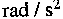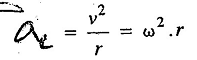# Angular Acceleration

What is Angular Acceleration, Centripetal Acceleration and Tangential Acceleration?

Angular Acceleration is the rate of change of angular velocity. It is usually expressed inand is denoted by a Greek letter α (alpha). It is also a vector quantity.

Notes : 1. If the body is moving along a circular path of radius r and with angular acceleration α, then its linear acceleration is given by

a = αxr

2. When a body moves along a circular path, its linear acceleration will have two components; one is the normal component and other is tangentia1 component. The normal component of the acceleration is known as normal or centripetal acceleration (ac). Its value is given by3. When the body moves along a circular path with uniform velocity, then there will be no tangential acceleration, but it will have only centripetal acceleration.

4. When the body moves along a straight path, then there will be no centripetal acceleration, but it will have only tangential acceleration, in the same direction as its velocity and displacement.